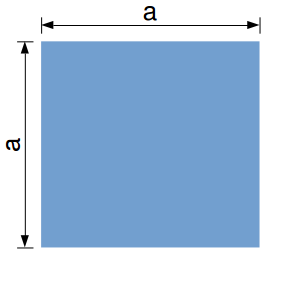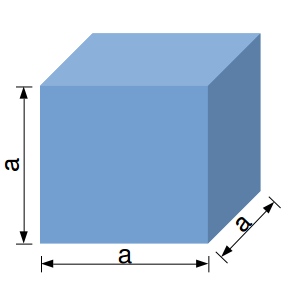# Exponentiation – integer exponents

In mathematics quite often it happens to add the same number several times:

$\begin{equation*} \begin{split} 4 + 4 + 4 + 4 + 4 \end{split} \end{equation*}$

This sum can be replaced by the product:

$\begin{equation*} \begin{split} 4 \cdot 5 \end{split} \end{equation*}$

In the same way we can encounter the multiple products of a number with itself:

$\begin{equation*} \begin{split} 4 \cdot 4\cdot 4\cdot 4\cdot 4 \end{split} \end{equation*}$

For this there is a short notation which is the “power” function or the exponentiation:

$\begin{equation*} \begin{split} 4^5 \end{split} \end{equation*}$

When we are speaking about a number raised at the “power” of another number we are using integer exponents.

For example, when we say “4 raised at the power of 2” we mean:

$\begin{equation*} \begin{split} 4^2 = 4 \cdot 4 = 16 \end{split} \end{equation*}$

To give the general definition of exponentiation we need two numbers, x and n. The number x raised at the power of n is written as:

$\begin{equation} \begin{split} \bbox[#FFFF9D]{x^n = \underbrace{x \cdot x \cdot … \cdot x}_\text{n times}} \end{split} \end{equation}$

With this in mind, we can define exponentiation as a mathematical operation with two numbers, a base x and an exponent n. When the exponent x is integer and positive, exponentiations means multiplying n times the base x.

Example:

$\begin{equation*} \begin{split} 4^5 = 4 \cdot 4 \cdot 4 \cdot 4 \cdot 4 = 1024 \end{split} \end{equation*}$

In this article we are going to focus on the properties of integer exponents, positive or negative. Also, exponentiation makes sense only if the base is different than zero. Raising zero at any power is not defined (doesn’t make sense) in mathematics.

The properties of integer exponents are listed below:

 $\begin{equation*} \begin{split} x^n \cdot x^m =& x^{n+m}\\ (x^n)^m =& x^{n \cdot m}\\ \frac{x^n}{x^m} =& x^{n-m}\\ (x \cdot y)^n =& x^n \cdot y^n\\ \left ( \frac{x}{y} \right )^n =& \frac{x^n}{y^n}\\ \left ( \frac{x}{y} \right )^{-n} =& \left ( \frac{y}{x} \right )^{n} = \frac{y^n}{x^n}\\ \frac{1}{x^{-n}} =& x^n\\ \left ( x \cdot y \right )^{-n} =& \frac{1}{\left ( x \cdot y \right )^n}\\ \frac{x^{-n}}{y^{-m}} =& \frac{y^m}{x^n}\\ \left ( x^n \cdot y^m \right )^k =& x^{n \cdot k} \cdot y^{m \cdot k}\\ \left ( \frac{x^n}{y^m} \right )^k =& \frac{x^{n \cdot k}}{y^{m \cdot k}} \end{split} \end{equation*}$

When using exponentiation we need to keep in mind two special cases:

• every number raised at the power of 0 equals 1
• every number raised at the power of 1 equals the same number
$\begin{equation} \begin{split} \bbox[#FFFF9D]{x^0 = 1}\\ \bbox[#FFFF9D]{x^1 = x} \end{split} \end{equation}$

Examples:

$\begin{equation*} \begin{split} 4^0 = 1\\ 4^1 = 4 \end{split} \end{equation*}$

It is also very important to keep in mind the importance of the parenthesis used for exponentiation. For example:

$\begin{equation*} \begin{split} (-4)^2 = 16 \hspace{10 mm}\text{is different than}\hspace{10 mm} -(4)^2 = -16 \end{split} \end{equation*}$

The general rule is that any negative number –x raised at an even power 2k will result in a positive number:

$\begin{equation} \begin{split} \bbox[#FFFF9D]{\left ( -x \right)^{2 \cdot k} = x^{2 \cdot k}} \end{split} \end{equation}$

For a better understanding on integer exponents we’ll do a couple of exercises in which we have to simplify mathematical expressions using the properties of exponentiation.

Exercise 1:

$\begin{equation*} \begin{split} \left (3 \cdot x^2 \cdot y^{-4} \right)^2 = 3^2 \cdot x^4 \cdot y^{-8} \end{split} \end{equation*}$

Exercise 2:

$\begin{equation*} \begin{split} \frac{7 \cdot x \cdot y^2}{\left (2 \cdot y \right)^{-3} \cdot x^4} =& \frac{7 \cdot x}{x^4} \cdot \frac{y^2}{2^{-3} \cdot y^{-3}}\\ =& 7 \cdot x^{1-4} \cdot \frac{1}{2^{-3}} \cdot y^{2+3}\\ =& \frac{7}{2^{-3}} \cdot x^{-3} \cdot y^5 \end{split} \end{equation*}$

Exercise 3:

$\begin{equation*} \begin{split} \left (4 \cdot x^3 \cdot y^{-2}\right) \cdot \left (-3 \cdot x^{-1} \cdot y^3\right)^{-2} =& \left (4 \cdot x^3 \cdot y^{-2}\right) \cdot \left (3^{-2} \cdot x^2 \cdot y^{-6}\right)\\ =& \frac{4}{3^2} \cdot x^5 \cdot y^{-8} \end{split} \end{equation*}$

Example 4:Square area: length (a) x height (a)

In geometry we calculate the area of a square as the product between length and width. Since they are equal, the are of the square becomes a product between the same number:

$\begin{equation*} \begin{split} A_{square} = a \cdot a = a^2 \end{split} \end{equation*}$Cube volume: width (a) x length (a) x height (a) x

If we want to calculate the volume of a cube we have to multiply the area with the height of the cube. This means that we have the product of the same number three times:

$\begin{equation*} \begin{split} V_{cube} = A_{square} \cdot a = a \cdot a \cdot a = a^3 \end{split} \end{equation*}$

This is a simple example which highlights how to use integer exponents to shorten the equations for square area and cube volume.

If you have any questions or observation regarding exponentiation with integer exponents use the comments section below. Don’t forget to Like, Share and Subscribe!

#### Ad Blocker Detected

Dear user, Our website provides free and high quality content by displaying ads to our visitors. Please support us by disabling your Ad blocker for our site. Thank you!